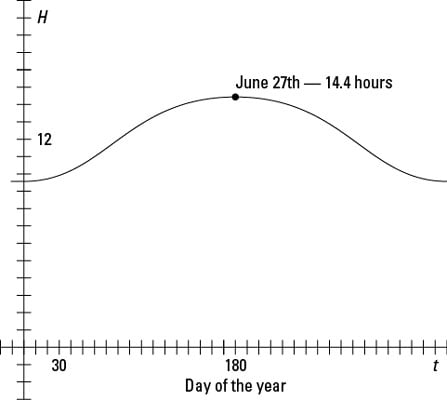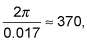##### Trigonometry For DummiesThe graphs of sine curves and the cofunction, cosine, are useful for modeling situations that happen over and over again in a predictable fashion. Some examples include the weather, seasonal sales of goods, body temperature, the tide’s height in a harbor, average temperatures, and so on.

Here’s an example: San Diego, California, is a gorgeous part of the world. Whether it’s summer or winter, you want to be there. But what if you’re someone who likes long, sunny days? When is the best time to go there?

Assume that the following formula gives you the number of hours of daylight in San Diego when you input any day of the year. Letting t be the day of the year (from 1 to 365), you can figure the number of hours of sunlight, H, if you enter a value for t in the equation H(t) = 2.4 sin (0.017t – 1.377) + 12. The following figure shows the graph of this equation.The number of hours of sunlight, H, in San Diego on Day t.

The amplitude of the sine curve is 2.4, which means that the number of daylight hours extends 2.4 hours above and below the average number of daylight hours. The average number of daylight hours is 12, which is the translation upward, meaning that the hours of sunlight range from 14.4 to 9.6, depending on the time of year. The period iswhich is a little longer than a year because of the rounding in the formula. The coefficient on the t means that 0.017 of the curve takes up the usual amount of space for one curve, 2π units. As you can see on the graph, the day with the most sunlight is June 27. You can determine that high point by using a graphing calculator that finds it for you — as well as the y-value of when t equals 14.4, or you can use calculus to solve it! Do you know, now, when you want to go to San Diego?